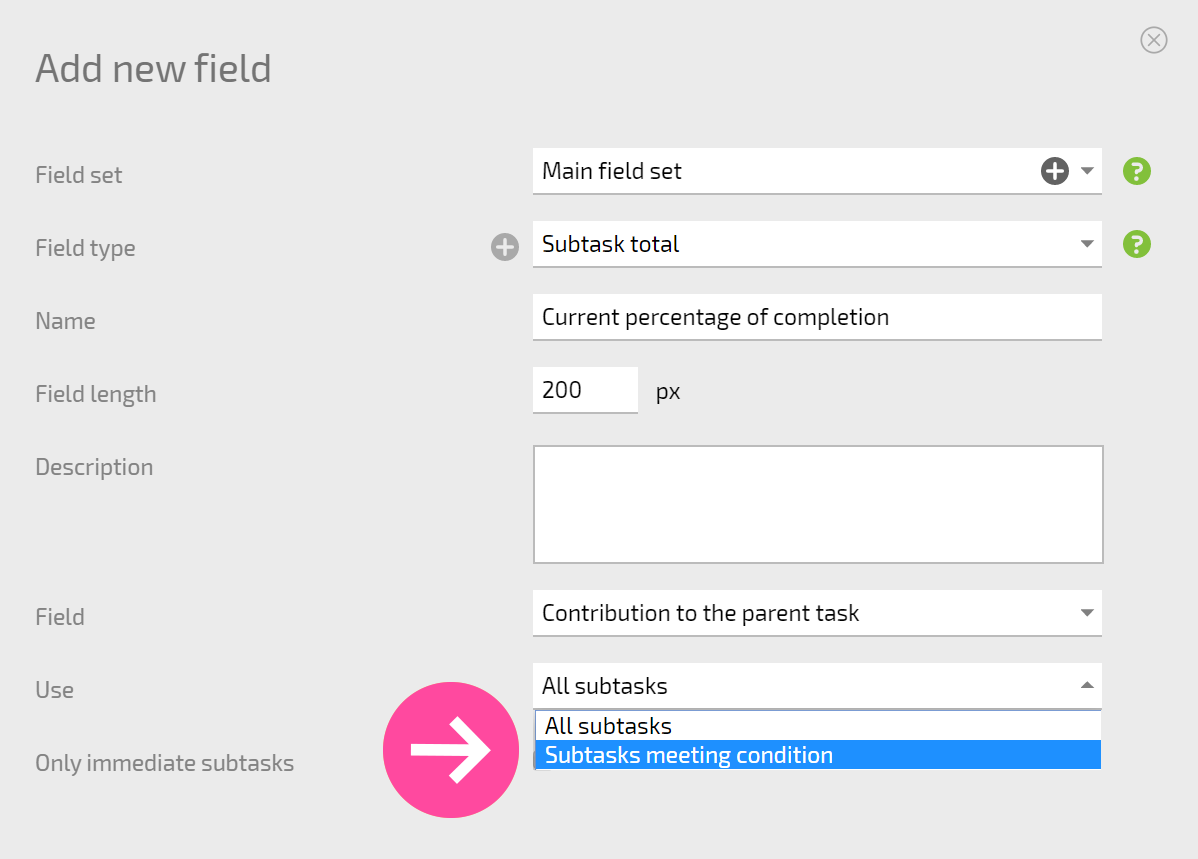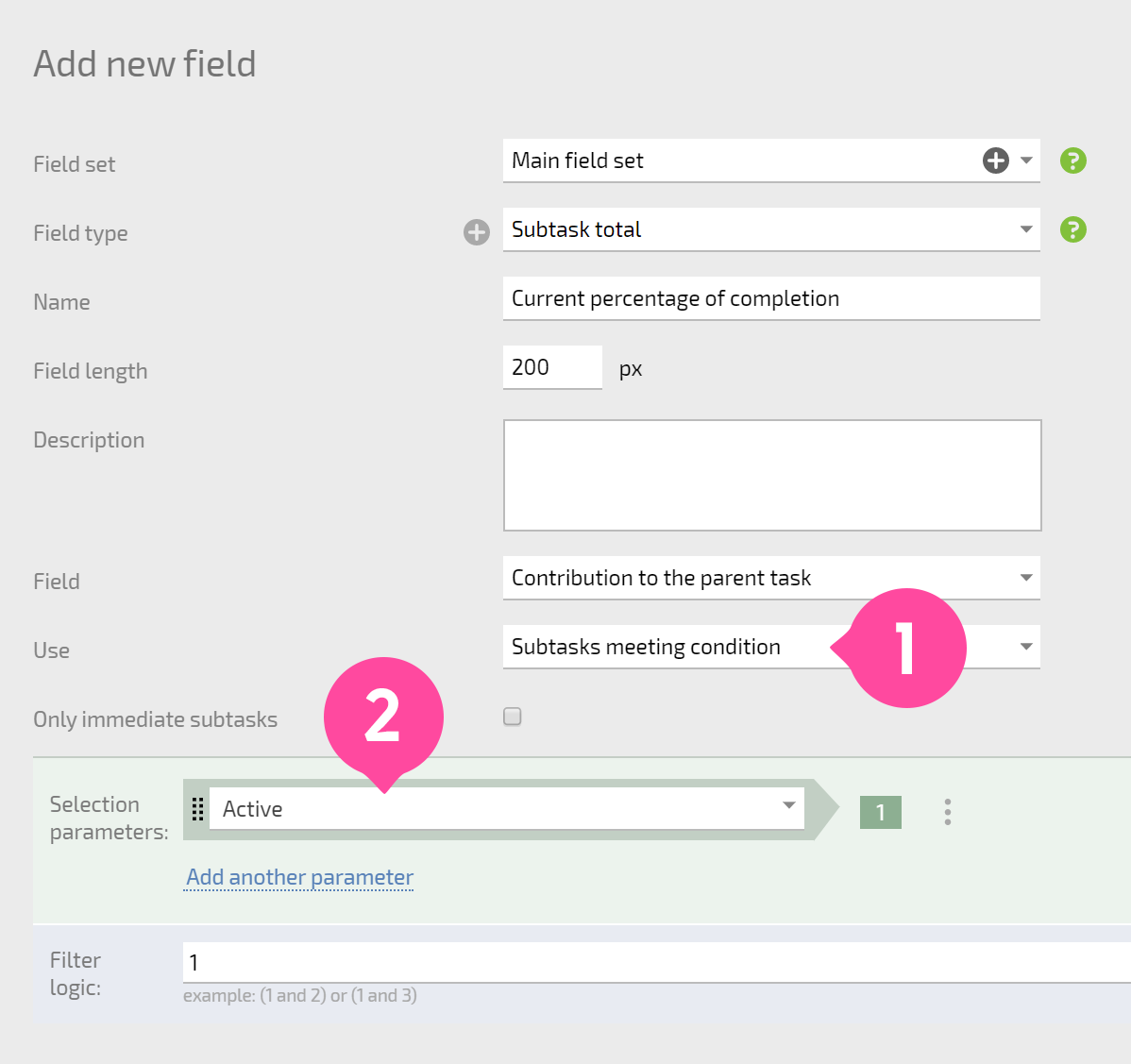Fields of this type are used to calculate and store total data for the selected subtask field. When a subtask field is modified and the field is part of a calculation, this causes the recalculation of the Subtask total fields in all of the parent tasks.

## Configuration

• The simplest option when configuring a Subtask total field is to choose a subtask field to use for the summation:You can also limit the subtasks where summation will happen by using a set of conditions. To do this, select the Subtasks meeting condition:For example, if you specify the condition in the screenshot below, the field will calculate the sum using only data from active tasks; data from completed, canceled, and other task statuses won't be counted: